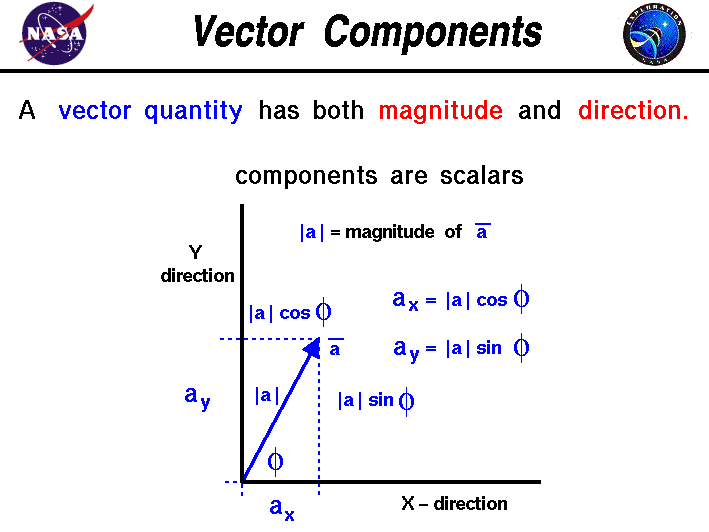+ Text Only Site
+ Non-Flash Version
+ Contact GlennMath and science were invented by humans to describe and understand the world around us. We observe that there are some quantities and processes in our world that depend on the direction in which they occur, and there are some quantities that do not depend on direction. Mathematicians and scientists call a quantity which depends on direction a vector quantity. A quantity which does not depend on direction is called a scalar quantity. A vector quantity has two characteristics, a magnitude and a direction. When comparing two vector quantities of the same type, you have to compare both the magnitude and the direction. On this slide we describe a mathematical concept which is unique to vectors; vector components. Vector components allow us to break a single vector quantity into two (or more) scalar quantities with which we have more mathematical experience. Vector components are used in vector algebra to add, subtract, and multiply vectors. Vectors are usually denoted on figures by an arrow. The length of the arrow indicates the magnitude of the vector and the tip of the arrow indicates the direction. The vector is labeled with an alphabetical letter with a line over the top to distinguish it from a scalar. We will denote the magnitude of the vector by the symbol |a|. The direction will be measured by an angle phi relative to a coordinate axis x. The coordinate axis y is perpendicular to x. Note: The coordinate axes x and y are themselves vectors! They have a magnitude and a direction. You first encounter coordinates axes when you learn to graph. So, you have been using vectors for some time without even knowing it! If we construct a dashed line from the tip of the vector a running parallel to the x-axis, it cuts the y-axis at a location we label ay. Similarly, a line from the tip of the vector parallel to the y-axis cuts the x-axis at ax. Using the sine and cosine relations from trigonometry: ay = |a| * sin(phi) ax = |a| * cos(phi) We call ax the x-component of a, and ay the y-component of a. The component equations are scalar equations; |a| and the trigonometric functions are just scalars. Any algebra involved with these quantities will be scalar algebra, not vector algebra. We have in essence replaced the single vector quantity a with two scalar quantities ax and ay. Looking very closely at these two equations, we notice that they completely define the vector quantity a; they specify both the magnitude and the direction of a. We can find the magnitude of the vector by using the Pythagorean Theorem. The components form two sides of a right triangle. To determine the length of the hypotenuse of the triangle: |a|^2 = ax^2 + ay^2 |a|^2 = |a|^2 sin^2(phi) + |a|^2 cos^2(phi) |a|^2 = |a|^2 (sin^2(phi) + cos^2(phi) ) |a|^2 = |a|^2 |a| = sqrt(|a|^2) Why go to all this trouble? Because, in aerospace, we are often dealing with forces and forces are vectors. Breaking a single vector force into several components allows us to study the resulting motion much more easily. Note: On this slide, for simplicity, we have developed the components in only two dimensions; there are two coordinate axes. In reality, there are three spatial dimensions and three components of all forces. This is important in our derivation of the general equations of motion for flight trajectories and for the Navier-Stokes and Euler equations which describe the forces and resulting motion of fluids in the engine. We can break very complex, three-dimensional, vector problems into only three scalar equations. Guided ToursVectors:Rocket Translation:Activities: Related Sites: Rocket Index Rocket Home Beginner's Guide Home+ Inspector General Hotline + Equal Employment Opportunity Data Posted Pursuant to the No Fear Act + Budgets, Strategic Plans and Accountability Reports + Freedom of Information Act + The President's Management Agenda + NASA Privacy Statement, Disclaimer, and Accessibility CertificationEditor: Tom Benson NASA Official: Tom Benson Last Updated: Jun 12 2014 + Contact Glenn